# Sorry

The easiest way of doing this problem is to first cross multiply or multiply both sides of the equation by the common denominator of both fractions.

Then divide both side by the coefficient of the unknown.

The original problem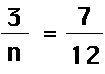Now multiply both side of the equation by the common denominator of both sides which in this case is 12n.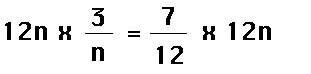Now do some cancelation before you multiply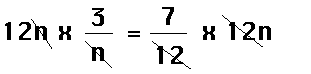Now multiply everything out. And you should get: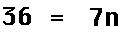Next divde both sides by the coefficient of the unknown, in this case the 7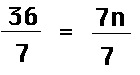Now do some cancelation.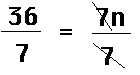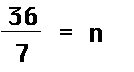All that remains now is to convert the 36/7 to a mixed fraction.

36/7 converted to a mixed fraction is 5 1/7.

Now let us try another one.

Solve for x.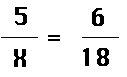<

Back to Proficiency Page